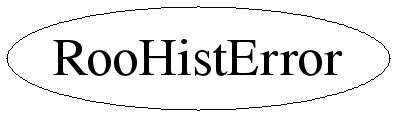# class RooHistError

## Function Members (Methods)

public:
 RooHistError(const RooHistError&) virtual ~RooHistError() static TClass* Class() static RooAbsFunc* createBinomialSum(Int_t n, Int_t m) static RooAbsFunc* createPoissonSum(Int_t n) Bool_t getBinomialInterval(Int_t n, Int_t m, Double_t& a1, Double_t& a2, Double_t nSigma = 1) const Bool_t getInterval(const RooAbsFunc* Qu, const RooAbsFunc* Ql, Double_t pointEstimate, Double_t stepSize, Double_t& lo, Double_t& hi, Double_t nSigma) const Bool_t getPoissonInterval(Int_t n, Double_t& mu1, Double_t& mu2, Double_t nSigma = 1) const static const RooHistError& instance() virtual TClass* IsA() const RooHistError& operator=(const RooHistError&) virtual void ShowMembers(TMemberInspector& insp, char* parent) virtual void Streamer(TBuffer& b) void StreamerNVirtual(TBuffer& b)
private:
 RooHistError() Bool_t getPoissonIntervalCalc(Int_t n, Double_t& mu1, Double_t& mu2, Double_t nSigma = 1) const Double_t seek(const RooAbsFunc& f, Double_t startAt, Double_t step, Double_t value) const

## Data Members

private:
 Double_t _poissonHiLUT Double_t _poissonLoLUT

## Class Charts## Function documentation

const RooHistError & instance()
``` Return a reference to a singleton object that is created the
first time this method is called. Only one object will be
constructed per ROOT session.
```
RooHistError(const RooHistError& )
``` Construct our singleton object.
```
Bool_t getPoissonInterval(Int_t n, Double_t& mu1, Double_t& mu2, Double_t nSigma = 1) const
``` Use lookup table for most common cases
```
Bool_t getPoissonIntervalCalc(Int_t n, Double_t& mu1, Double_t& mu2, Double_t nSigma = 1) const
``` Calculate a confidence interval for the expected number of events given n
observed (unweighted) events. The interval will contain the same probability
as nSigma of a Gaussian. Uses a central interval unless this does not enclose
the point estimate n (ie, for small n) in which case the interval is adjusted
to start at n.
```
Bool_t getBinomialInterval(Int_t n, Int_t m, Double_t& a1, Double_t& a2, Double_t nSigma = 1) const
``` sanity checks
```
Bool_t getInterval(const RooAbsFunc* Qu, const RooAbsFunc* Ql, Double_t pointEstimate, Double_t stepSize, Double_t& lo, Double_t& hi, Double_t nSigma) const
``` Calculate a confidence interval using the cummulative functions provided.
The interval will be "central" when both cummulative functions are provided,
unless this would exclude the pointEstimate, in which case a one-sided interval
pinned at the point estimate is returned instead.
```
Double_t seek(const RooAbsFunc& f, Double_t startAt, Double_t step, Double_t value) const
``` Scan f(x)-value until it changes sign. Start at the specified point and take constant
steps of the specified size. Give up after 1000 steps.
```

virtual ~RooHistError()
`{}`
RooHistError(const RooHistError& )

Last update: Thu Jan 17 08:44:57 2008
Copyright (c) 2000-2005, Regents of the University of California *

This page has been automatically generated. If you have any comments or suggestions about the page layout send a mail to ROOT support, or contact the developers with any questions or problems regarding ROOT.